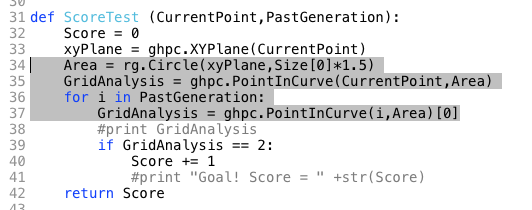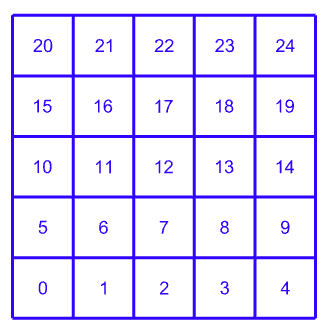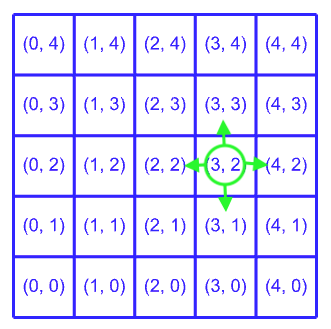# How can I optimize this Gh Python Code?

Hello,

I’m kind of new to coding, and as an excercise I wrote a Python script, which generates new generations of cubes, based on the Game Of Life rules.

The reason I’m posting this, is because it seems to be quite heavy, and I just want to understand a few trick to help optimize the performance of the definition (just for learning purposes).

Just a tip: Enable lock solver, because the definition takes around 30s to process.

And help or recomendation is welcomed!

GameOfLife V2.1.gh (10.1 KB)

Hi @Frusciante
I quickly scimmed through your code, and it caught my eye that you are generating and evaluating geometry methods (Point3D, Box, Circle, pointInCurve). Hard to say yet, but my assumption is that these are the culprits in slowing it down.

I suggest, instead of using geometries, just store coordinates - or even better keep track of neighbours through their respective location in the Array/Tree. I.e. if you know the grid size in X,Y & Z, you know the address to each neighbour.

1 Like

I found an old CellularAutomata script I’ve done in 2009 in Rhinoscript (you can just drag the script to Rhino window - or write “monkey” in Rhino).

There I’m using textDots to visualise the grid and just storing the living/dead information inside the array. The calculations are processed through the cell locations inside the array.

``````Option Explicit
'Script written by Toni Österlund <toni.osterlund@gmail.com>
'Script version Monday, 02 November 2009 23:34:03

Call Main()
Sub Main()
Call Rhino.EnableRedraw(False)

Dim Generations : Generations = 15
Dim SizeX, SizeY, SizeZ
SizeX = 7
SizeY = 7
SizeZ = 7

Dim arrDots : arrDots = CellularAutomata(Generations, SizeX, SizeY, SizeZ)

Dim CaState : CaState = Rhino.TextDotText(arrDots(2, 3, 0))
If CaState = 1 Then
Call Rhino.Print("Solu koordinaateissa 2,3,0 on elävä")
Else
Call Rhino.Print("Solu koordinaateissa 2,3,0 on kuollut")
End If

Call Rhino.EnableRedraw(True)
End Sub

Function CellularAutomata(ByVal intIterations, ByVal intSizeU, ByVal intSizeV, ByVal intSizeW)
CellularAutomata = Null

'-----------------------------------------------------------------------------------------------------
'set the rules, so it will scale the number of neightbours needed to live/die/rebirth
'1 layer (2D)  = 8   neighbours MAX (intSizeW = 1)
'2 layers (3d) = 17  neighbours MAX (intSizeW = 2)
'3+ layers (3d) = 26 neighbours MAX (intSizeW = 3+)
Dim arrRules(2)
If IntSizeW = 1 Then '2D
arrRules(0) = 2 'neighbours under this number, will kill the cell due to underpopulation
arrRules(1) = 3 'neighbours over this number, will kill the cell due to overpopulation
arrRules(2) = 3 'this many neighbours will reinstate the cell
Else '3D
arrRules(0) = 4
arrRules(1) = 9
arrRules(2) = 5
End If

'-----------------------------------------------------------------------------------------------------
'Rescale the grid to start from 0
'ie. gridsize = 1 -> would make 2 colums (0,1 => 2pcs)
intSizeU = intSizeU - 1
intSizeV = intSizeV - 1
intSizeW = intSizeW - 1

'-----------------------------------------------------------------------------------------------------
'calculate random values for each cell at start
'1 = living   0 = dead
Dim arrState, x,y,z
ReDim arrState(intSizeU, intSizeV, intSizeW)
Dim arrDots
For x = 0 To intSizeU
For y = 0 To intSizeV
For z = 0 To intSizeW

Call Randomize()
If Rnd < 0.3 Then
arrState(x, y, z) = 1
Else
arrState(x, y, z) = 0
End If

Next
Next
Next

Call Rhino.EnableRedraw(False)
'make the grid with the first set of random values
arrDots = Make3dGrid(arrState, Array(intSizeU, intSizeV, intSizeW))
Call Rhino.EnableRedraw(True)

'-----------------------------------------------------------------------------------------------------
'calculate the CA
Dim N
Dim ZLimit(1)
Dim p,q,r
Dim XX,YY,ZZ
Dim IntState, intNeighTotal

'for the amount of iterations
For N = 0 To intIterations
Call Rhino.EnableRedraw(False)
Call Rhino.Print(intIterations - N & " iterations left to calculate")

For x = 0 To intSizeU
For y = 0 To intSizeV
For z = 0 To intSizeW
'get the initial cell state
IntState = arrState(x, y, z)
intNeighTotal = 0

If intSizeW = 0 Then
ZLimit(0) = 0
ZLimit(1) = 0
Else
ZLimit(0) = -1
ZLimit(1) = 1
End If

'calculate the neighbours
For p = -1 To 1
For q = -1 To 1
For r = ZLimit(0) To ZLimit(1)
'if all are 0 then it is the basepoint, and its value will not be added
If p><0 Or q><0 Or r><0 Then

XX = x + p
YY = y + q
ZZ = z + r

'exceptions, if the point is located in the exterior layer
'then wrap the solution
If XX < 0 Then XX = intSizeU
If YY < 0 Then YY = intSizeV
If ZZ < 0 Then ZZ = intSizeW
If XX > intSizeU Then XX = 0
If YY > intSizeV Then YY = 0
If ZZ > intSizeW Then ZZ = 0

If Rhino.TextDotText(arrDots(XX, YY, ZZ)) = 1 Then
intNeighTotal = intNeighTotal + 1
End If
End If

Next
Next
Next
'calculate the cells new state
If intState = 1 Then 'if the cell is living
If intNeighTotal < arrRules(0) Then
arrState(x, y, z) = 0
ElseIf intNeighTotal > arrRules(1) Then
arrState(x, y, z) = 0
Else
arrState(x, y, z) = 1
End If
Else 'if the cell is dead (intState=0)
If intNeighTotal = arrRules(2) Then
arrState(x, y, z) = 1
Else
arrState(x, y, z) = 0
End If
End If

'Call Rhino.Print("cellstate=" & IntState & " - neighbours:" & intNeighTotal & " - new state:" & arrState(x, y, z))

Next
Next
Next

Call UpdateGrid(arrDots, arrState, Array(intSizeU, intSizeV, intSizeW)) 'update the grid with the newly calculated data
Call Rhino.EnableRedraw(True)
Next

CellularAutomata = arrDots
End Function

Sub UpdateGrid(ByRef arrDots, ByRef arrValues, ByRef arrSize)
Dim x, y, z
For x=0 To arrSize(0)
For y=0 To arrSize(1)
For z=0 To arrSize(2)
Call Rhino.TextDotText(arrDots(x, y, z), arrValues(x, y, z))
If arrValues(x, y, z) = 1 Then
Call Rhino.ObjectColor(arrDots(x, y, z), vbwhite)
Else
Call Rhino.ObjectColor(arrDots(x, y, z), vbblack)
End If
Next
Next
Next

End Sub

Function Make3dGrid(ByRef arrValues, ByRef arrSize)
Make3dGrid = Null

Dim calc : calc = 0
Dim x, y, z
Dim arrDots()
ReDim arrDots(arrSize(0), arrSize(1), arrSize(2))

For x=0 To arrSize(0)
For y=0 To arrSize(1)
For z=0 To arrSize(2)
arrDots(x, y, z) = Rhino.AddTextDot(arrValues(x, y, z), array(x, y, z))
If arrValues(x, y, z) = 1 Then
Call Rhino.ObjectColor(arrDots(x, y, z), vbwhite)
Else
Call Rhino.ObjectColor(arrDots(x, y, z), vbblack)
End If
Next
Next
Next

Make3dGrid = arrDots
End Function
``````

CellularAutomata.rvb (5.7 KB)

1 Like

Hi,

Back in 2019, I posted a dedicated GHPython component for the Game of Life.
You can find it here:

The code is open-source, if you want to take a peek.

1 Like

Concerning your code, I guess you could speed it up a lot, by getting rid of stuff like this:The issue is that you’re performing hundreds or even thousands of point in curve searches at each iteration of the game, which is very expensive and unnecessary.

Let’s say you have a simple square grid, where each cell is indexed, has side length 1, and has an on/off state. It’s really all you need, and can be a simple list with booleans:Now let’s say you want to get the normalized (x, y) coordinates of the cell at index 13.

`row = floor(index / number of columns) = 13 / 5 = 2`
`column = index % number of columns = 13 % 5 = 3`

Since, the initial grid is normalized, meaning each cell is 1 by 1 units in size, you need to multiply the row and column values by the real side length to get the bottom left cell corner point.

You can also get the index of a cell from its normalized (x, y) coordinates:

`index = column + row * number of columns = 3 + 2 * 5 = 13`

Now, to get a cell neighbour, all you really have to do is subtract or add one unit to the row and/or column your currently at. Here the benefit of the normalized grid is clearly discernable.`northern neighbour = (column, row + 1) = (3, 2 + 1) = (3, 3); if row + 1 < number of rows`
`eastern neighbour = (column + 1, row) = (3 + 1, 2) = (4, 2); if column + 1 < number of columns`
`southern neighbour = (column, row - 1) = (3, 2 - 1) = (3, 1); if row - 1 >= 0`
`western neighbour = (column - 1, row) = (3 - 1, 2) = (2, 2); if column - 1 >= 0`

Once you have the normalized (x, y) coordinates of a neighbour, you can again get its index and thus state by simply applying the above formula:

`western neighbour index = 2 + 2 * 5 = 12`

3 Likes

Interesting… I think this is exactly what I’m looking for.

It’s dificult, as an architect, to stop looking at problem solving through a more math oriented solution, instead of a visual/geometric approach.

I will definitely dive into this method to optimize this script. And take it into consideration for future projects.

Thank you!!

Arrays are something I really need to dive into. Initially my intentions where to learn Arrays before doing this project, but seems like I couldn’t help myself.

I have tried your code, although rules are different, the time it takes to process seems very optimized.

Thanks you for this!

1 Like